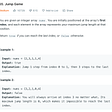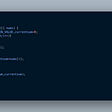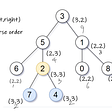# Leetcode — 5. Longest Palindromic Substring (中文)

`範例Input: s = "babad"Output: "bab" -> 因s字串中, 最長可組成的回文子字串長度是3, 答案回傳"aba"也算對`

# 想法:

🔧方法1. 我們把pointer i放在字串的第一個字元, pointer j放在字串的最後一個字元, 在比對完i和j指的字元後, pointer i跟pointer j都將會往中間移動, 如果這個字串是回文的話, 那pointer i和pointer j指的字元都會一樣, 直到i和j在字串最中間兩點相遇或交錯

🔧方法2. 這次我們pointer i和pointer j的起始點在字串中間, 分別讓i往字串的第一個字走(也就是往左走到底), 讓j往字串最後一個字走(也就是往右走到底), 如同方法1, pointer i和pointer j在走到底前, 他們兩個指的字元也應該要一模一樣, 整個字串才能被稱為是回文

⚠️在我們的helper method中, 我們用方法1或方法2來解都沒有插, 畢竟這兩個方法時間複雜度是一樣的

`public String longestPalindrome(String s) {        int n = s.length();        if(n<=1)            return s;        for(int len=n;len>=2;len--)        {            for(int left=0;left+len<=n;left++)            {                int right = left+len-1;                if(isPal(s,left,right))                    return s.substring(left, right+1);            }        }        return s.substring(0,1);    }    public boolean isPal(String s, int l,int r){        while(l<r)        {            if(s.charAt(l++)!=s.charAt(r--))                return false;        }        return true;    }`

`比如說, 假設我們已知“acbca”是回文了那如果我們想知道“xacbcax”是否為回文, 我們只要比對第一個字元跟最後一個字元是否相同, 若相同, 就可以直接判斷“xacbcax”就跟它裡面的子字串“acbca”一樣, 也是回文`

`public String longestPalindrome(String s) {        int n = s.length();        if(n<=1)            return s;        String rst = s.substring(0,1);        boolean[][] dp = new boolean[n][n];        for(int len=1;len<=n;len++)        {            for(int left=0;left+len<=n;left++)            {                int right = left+len-1;                if(s.charAt(left)!=s.charAt(right))                    continue;                if(right-left<=2)//like "a" "aa" "aba"                    dp[left][right] = true;                else                    dp[left][right] = dp[left+1][right-1];                int curLen = rst.length();                if(dp[left][right] && curLen < len)                    rst = s.substring(left,right+1);            }        }        return rst;    }`

# 這次, 我們不猜最終子字串的起點和終點了, 我們這次改成猜最終子字串的中間點

`舉例來說, 假設字串為"abcde"那假設我們要找b為中間點的子字串, 那b為中間點所能組成的最長子字串就是"abc"和"abcd"`

`public String longestPalindrome(String s) {        String rst = "";        int n = s.length();        for(int i=0;i<n;i++)        {            //i is the mid point of the substr            String cur = helper(s, i-1, i+1);            if(cur.length()>rst.length())                rst = cur;            cur = helper(s, i, i+1);            if(cur.length()>rst.length())                rst = cur;        }        return rst;    }    public String helper(String s, int left,int right){        int n = s.length();        while(left>=0 && right<n               && s.charAt(left) == s.charAt(right))        {            left--;            right++;        }        //here: s[left] != s[right]        return s.substring(left+1, right);    }`

# 因此, 若我們發現現在位置i根本不可能組成比現在答案字串還長的子字串的時候, 我們可以直接不去呼叫helper method, 去找下一個

`public String longestPalindrome(String s) {        String rst = "";        int n = s.length();        for(int i=0;i<n;i++)        {            int longest = Math.min(i, n-i+1);            if(rst.length() > longest*2)                return rst;            //i is the mid point of the substr            String cur = helper(s, i-1, i+1);            if(cur.length()>rst.length())                rst = cur;            cur = helper(s, i, i+1);            if(cur.length()>rst.length())                rst = cur;        }        return rst;    }    public String helper(String s, int left,int right){        int n = s.length();        while(left>=0 && right<n               && s.charAt(left) == s.charAt(right))        {            left--;            right++;        }        //here: s[left] != s[right]        return s.substring(left+1, right);    }`

--

--

--

## More from Anj

Click here to view all the stories posted on this blog: https://anj910.medium.com/bookmark-c95e4c52cf44

Love podcasts or audiobooks? Learn on the go with our new app.## Anj

Click here to view all the stories posted on this blog: https://anj910.medium.com/bookmark-c95e4c52cf44

## Jump Game: Leetcode Medium — Blind 75 (Greedy)## Largest Sum Contiguous Sub-Array## The Essence of Algorithm: Lowest Common Ancestor